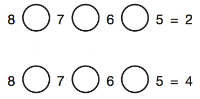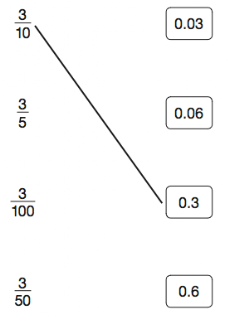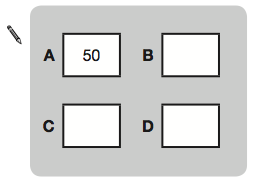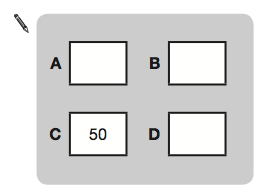# P7 Maths Homework – Revision 2Please complete the following questions in your Maths Homework jotter for Friday 12th May. Remember to write the date, title and show all of your working and write a sentence to show your final answer, where appropriate.
1. Copy and complete the following calculations by using a + or a –2. Megan has 7 coins that make one pound. The coins are of only two different kinds. What are the coins?

3. The numbers in this sequence increase by 10 each time. 3, 13, 23… The sequence continues in the same way.

a) Write two numbers from the sequence that add to make a total of 96.

b) Explain why it is not possible to find three numbers from the sequence that add to make a total of 96.

4. Match each fraction to the correct decimal number. The first one has been done for you.5. Here are four shapes on a square grid. Write the letters of all the shapes that have exactly two sides which are equal in length.6. Calculate 332.18 minus 7.062

7. Copy and complete the following sentences by choosing one of the options:

a) The length of a banana is about… 2cm, 20cm, 2mm, 2m, 20m.

b) The mass of an apple is about… 2g, 20kg, 200kg, 200g, 2kg.

c) A glass of fruit juice is about… 2ml, 2l, 20ml, 200ml, 20l.

8. a) The number in A is twice the number in D.

The number is B is 5 less than the number in C.

The number in D is 10 more than the number in B.b) Now use the same rule for this diagram.9.  200 children went on holiday. 10% of the children went to Wales. 25% of the children went to Scotland. How many more children went to Scotland than went to Wales?

10. Amy did a survey of what time people get up on a Sunday morning. This table shows her results for 150 people.a) How many people get up at 8am or later?

b) Amy says, “Two-thirds of the 150 people in the survey get up before 9am.” Is Amy correct? SHow your working to explain your answer.

11. Copy and complete the calculation to make it correct.12. This graph shows how the temperature changed in Liam’s room one afternoon.a) Estimate the temperature at 3.15pm.

b) Estimate the time when the temperature was highest.

c) How much did the temperature change from 2pm to 2.30pm? Give your answer to the nearest degree.

13. Alfie has two sticks. He puts them end to end.

One stick is 10cm longer than the other stick. How long are the two sticks?

14. Megan and Chen are washing cars. Megan earns £39 and Chen gets £55. They share what they get equally between them. How much does each of them get?

15. Four children are in a race. Chen is two metres ahead of Alfie. Nina is 5 metres behind Megan. Alfie is three metres behind Megan. Write the names of the runners in order, starting with the child who is furthest ahead.